# wishgale / awesome_lightweight_networks

The implementation of various lightweight networks by using PyTorch. such as：MobileNetV2，MobileNeXt，GhostNet，AdderNet，ShuffleNetV1-V2，LCNet，Mobile+ViT etc. ⭐⭐⭐⭐⭐

https://github.com/murufeng/awesome_lightweight_networks

Geek Repo

Github PK Tool

# awesome_lightweight_networks### 安装

`pip install light_cnns`

### Bag of Tricks for Image Classification with Convolutional Neural Networks

#### 网络结构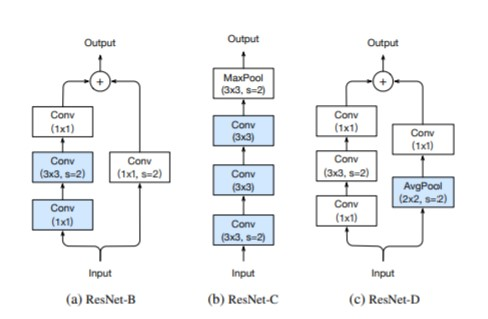#### Code

```import torch
from light_cnns import resnet50_v1b
model = resnet50_v1b()
model.eval()
print(model)
input = torch.randn(1, 3, 224, 224)
y = model(input)
print(y.size())```

### MobileNets系列

#### MobileNetv1 网络模块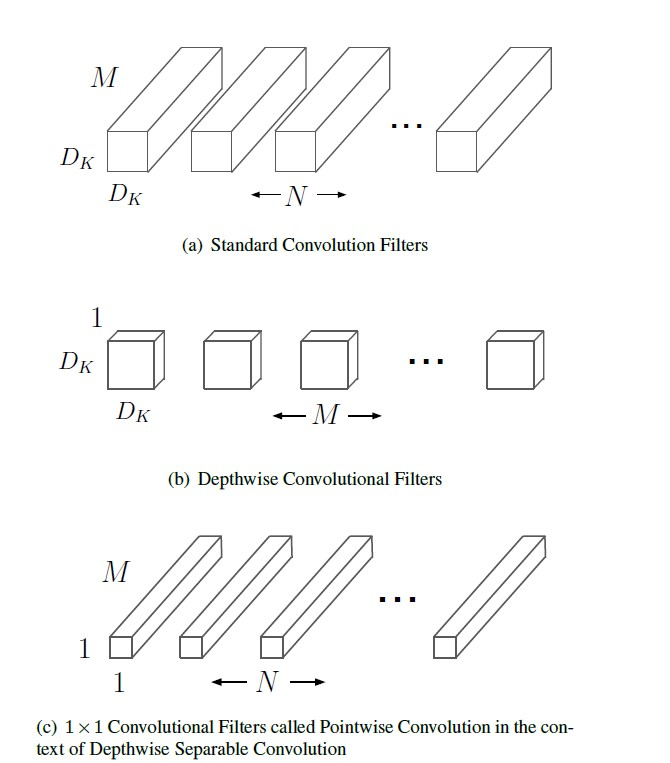#### Code

```import torch
from light_cnns import mbv1
model = mbv1()
model.eval()
print(model)
input = torch.randn(1, 3, 224, 224)
y = model(input)
print(y.size())```

#### MobileNetv2 网络模块

mobilenetv2 沿用特征复用结构（残差结构），首先进行Expansion操作然后再进行Projection操作，最终构建一个逆残差网络模块（即Inverted residual block）。

• 增强了梯度的传播，显著减少推理期间所需的内存占用。
• 使用 RELU6（最高输出为 6）激活函数，使得模型在低精度计算下具有更强的鲁棒性。
• 在经过projection layer转换到低维空间后，将第二个pointwise convolution后的 ReLU6改成Linear结构，保留了特征多样性，增强网络的表达能力（Linear Bottleneck）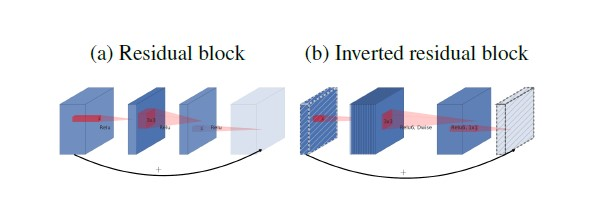#### Code

```import torch
from light_cnns import mbv2
model = mbv2()
model.eval()
print(model)
input = torch.randn(1, 3, 224, 224)
y = model(input)
print(y.size())```

#### MobileNetV3 网络模块

Searching for MobileNetV3

• 网络的架构基于NAS实现的MnasNet（效果比MobileNetV2好）
• 论文推出两个版本：Large 和 Small，分别适用于不同的场景;
• 继承了MobileNetV1的深度可分离卷积
• 继承了MobileNetV2的具有线性瓶颈的倒残差结构
• 引入基于squeeze and excitation结构的轻量级注意力模型(SE)
• 使用了一种新的激活函数h-swish(x)
• 网络结构搜索中，结合两种技术：资源受限的NAS（platform-aware NAS）与NetAdapt 算法获得卷积核和通道的最佳数量

#### Code

```import torch
from light_cnns import mbv3_small
#from light_cnns import mbv3_large
model_small = mbv3_small()
#model_large = mbv3_large()
model_small.eval()
print(model_small)
input = torch.randn(1, 3, 224, 224)
y = model_small(input)
print(y.size())```

#### MobileNeXt 网络模块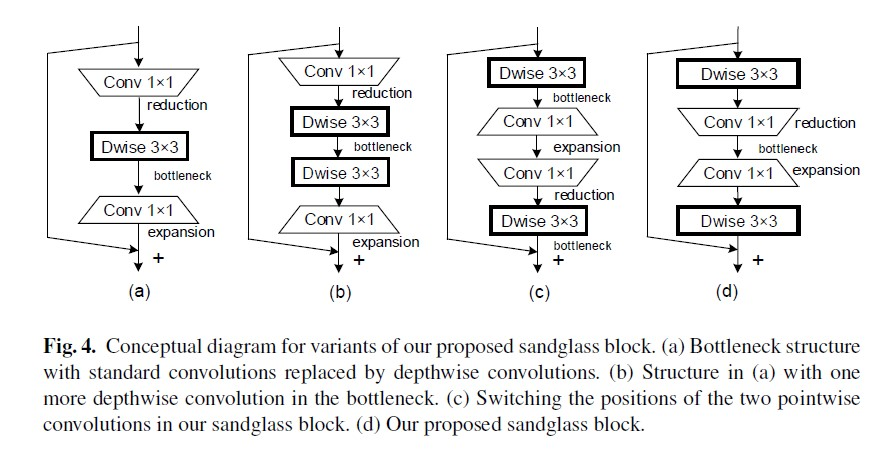#### Code

```import torch
from light_cnns import mobilenext
model = mobilenext()
model.eval()
print(model)
input = torch.randn(1, 3, 224, 224)
y = model(input)
print(y.size())```

#### ShuffleNetv1 网络模块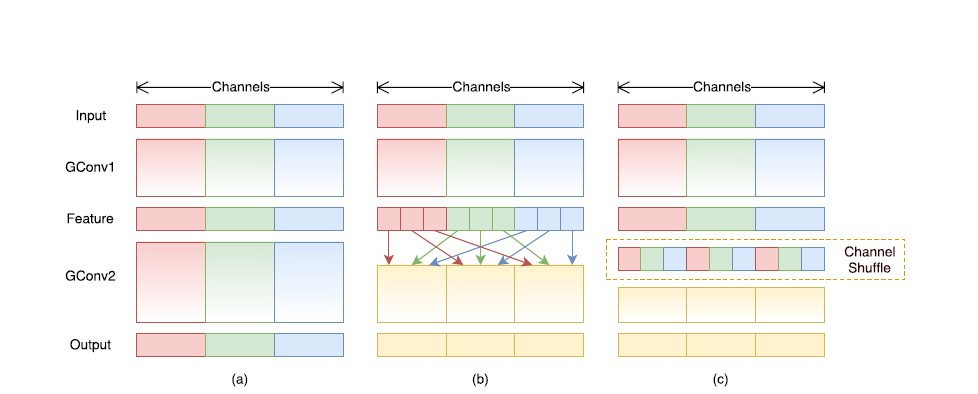#### Code

```import torch
from light_cnns import shufflenetv1
model = shufflenetv1()
model.eval()
print(model)
input = torch.randn(1, 3, 224, 224)
y = model(input)
print(y.size())```

#### ShuffleNetv2 网络模块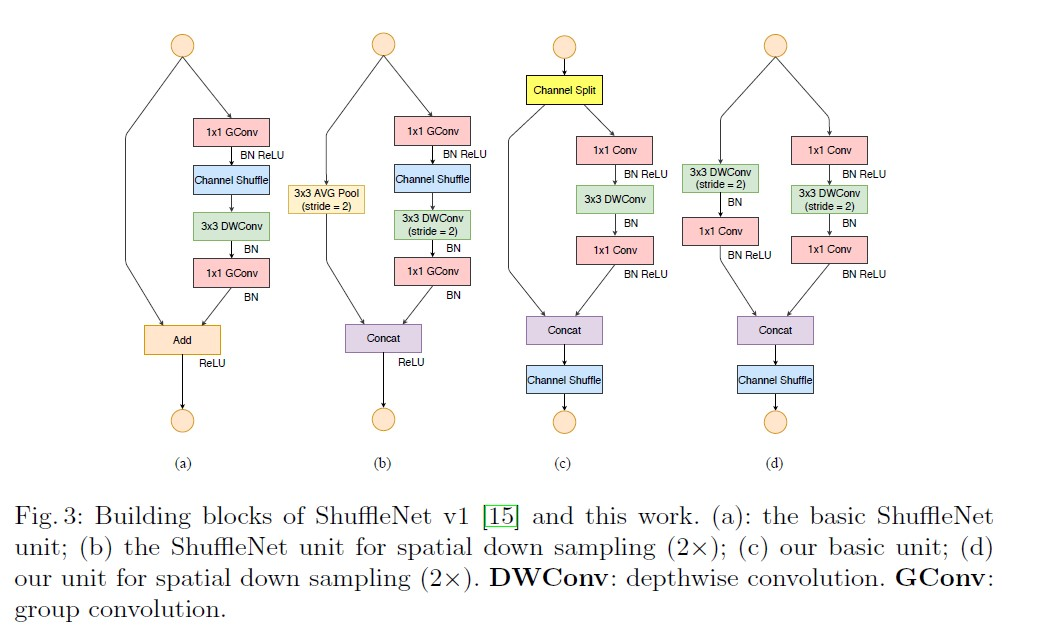#### Code

```import torch
from light_cnns import shufflenetv2
model = shufflenetv2()
model.eval()
print(model)
input = torch.randn(1, 3, 224, 224)
y = model(input)
print(y.size())```

### 华为诺亚方舟系列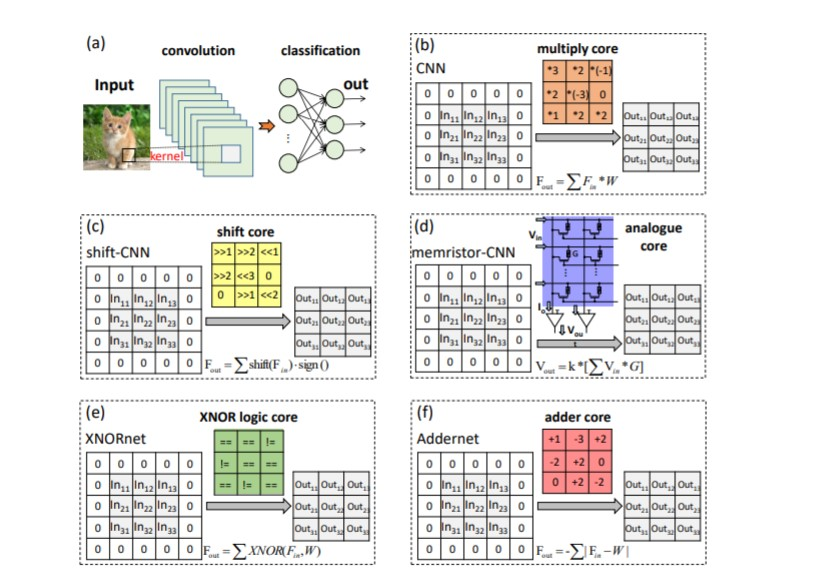#### Code

```import torch
from light_cnns import resnet20
model = resnet20()
model.eval()
print(model)
input = torch.randn(1, 3, 224, 224)
y = model(input)
print(y.size())```

#### GhostNet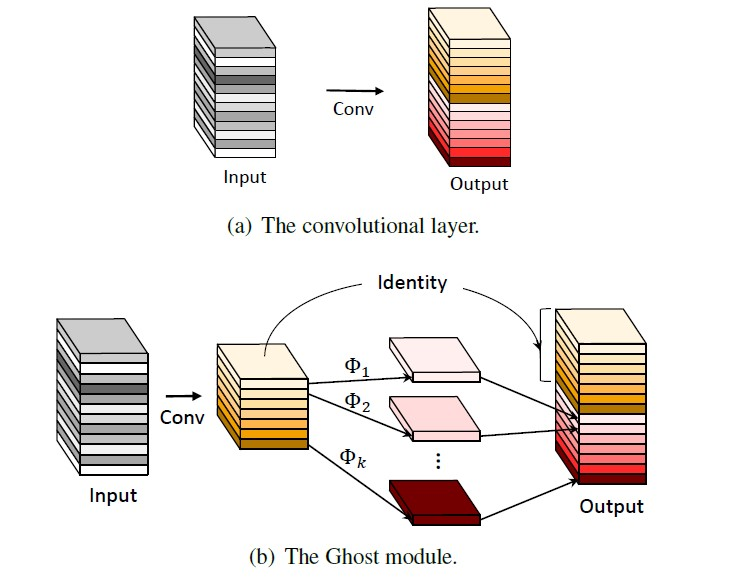#### Code

```import torch
from light_cnns import ghostnet
model = ghostnet()
model.eval()
print(model)
input = torch.randn(1, 3, 224, 224)
y = model(input)
print(y.size())```

### 注意力系列

#### CANet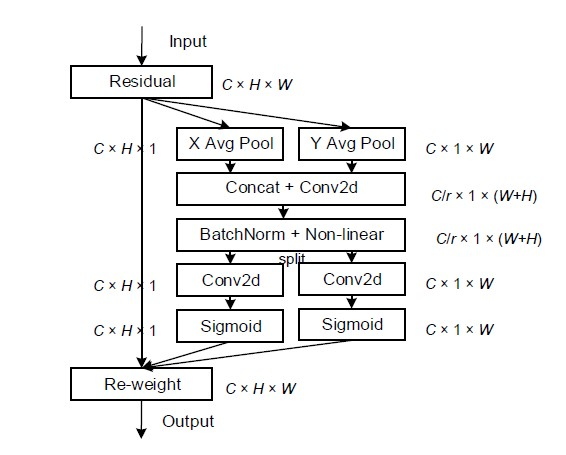#### Code

```import torch
from light_cnns import mbv2_ca
model = mbv2_ca()
model.eval()
print(model)
input = torch.randn(1, 3, 224, 224)
y = model(input)
print(y.size())```

#### ECANet

• ECA-Net: Efficient Channel Attention for Deep Convolutional Neural Networks

ECANet是一种即插即用的轻量级通道注意力模块，可显著提高CNN性能！ECANet主要对SENet模块进行了一些改进，提出了一种不降维的局部跨信道交互策略（ECA模块）和自适应选择一维卷积核大小的方法，该模块只增加了少量的参数，却能获得明显的性能增益。通过对SENet中通道注意模块的分析，实验表明避免降维对于学习通道注意力非常重要，适当的跨信道交互可以在显著降低模型复杂度的同时保持性能。因此，作者提出了一种不降维的局部跨信道交互策略，该策略可以通过一维卷积有效地实现。进一步，作者又提出了一种自适应选择一维卷积核大小的方法，以确定局部跨通道信息交互的覆盖率。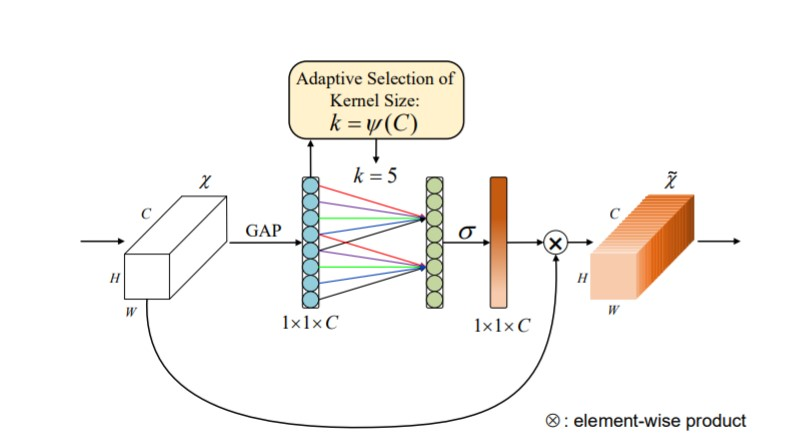• 代码实现
```import torch
from light_cnns import mbv2_eca
model = mbv2_eca()
model.eval()
print(model)
input = torch.randn(1, 3, 224, 224)
y = model(input)
print(y.size())```

#### ResNeSt

• ResNeSt: Split-Attention Networks

ResNeSt 实际上是站在巨人们上的"集大成者"，特别借鉴了：Multi-path 和 Feature-map Attention思想。作者@张航也提到了这篇文章主要是基于 SENet，SKNet 和 ResNeXt，把 attention 做到 group level。另外还引入了Split-Attention块，可以跨不同的feature-map组实现feature-map注意力。和其它网络主要的区别在于：

2. ResNeXt在ResNet bottle模块中采用分组卷积，将multi-path结构转换为统一操作。
3. SE-Net 通过自适应地重新校准通道特征响应来引入通道注意力（channel-attention）机制。
4. SK-Net 通过两个网络分支引入特征图注意力（feature-map attention）。一句话总结就是用multiple scale feature汇总得到总的feature map information，然后利用Softmax来指导channel-wise的注意力向量从而实现自适应分配不同尺度的表征信息。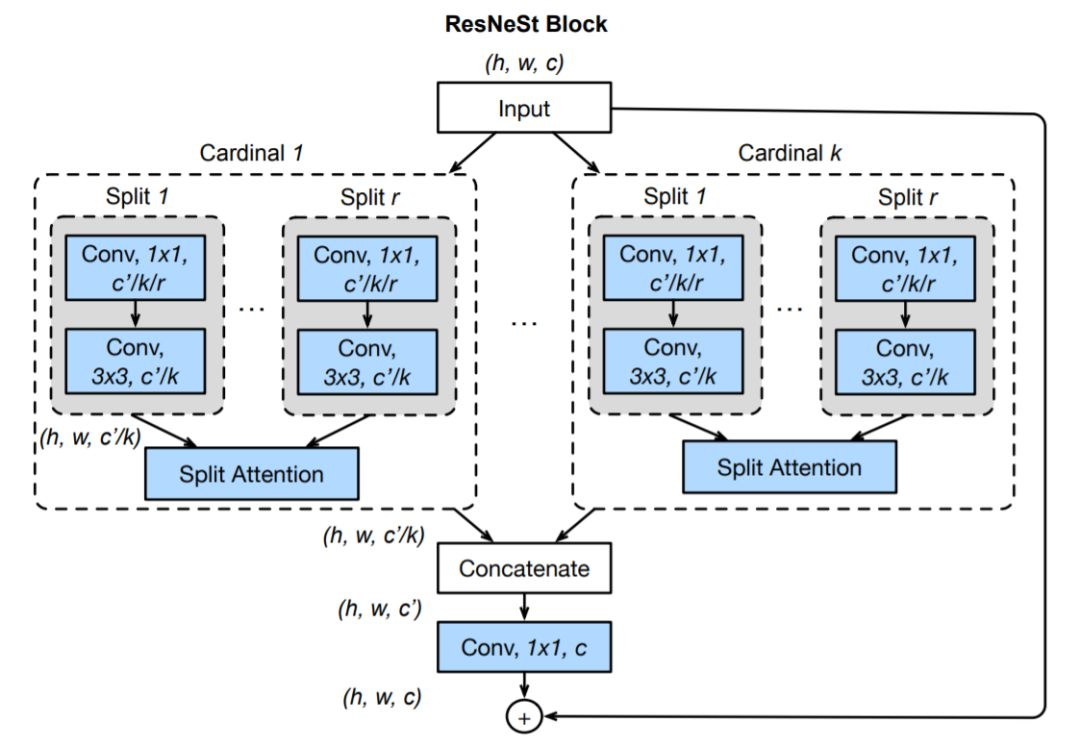• 代码实现
```import torch
from light_cnns import resnest50_v1b
model = resnest50_v1b()
model.eval()
print(model)
input = torch.randn(1, 3, 224, 224)
y = model(input)
print(y.size())```

#### SANet

• SA-Net: Shuffle Attention for Deep Convolutional Neural Networks

shuffle attention主要在空间注意力（Spatial Attention）与通道注意力（Channel Attention）的基础上，引入了特征分组与通道注意力信息置换这两个操作，得到了一种超轻量型的即插即用注意力模块。具体的说，SA首先将输入沿着通道维度拆分为多组，然后对每一组特征词用Shuffle unit 刻画与建模特征在空间维度与通道维度上的依赖关系，最后所有特征进行集成以及通过通道置换操作进行各组件单元的特征通信。主要结构如下所示：

• 网络结构：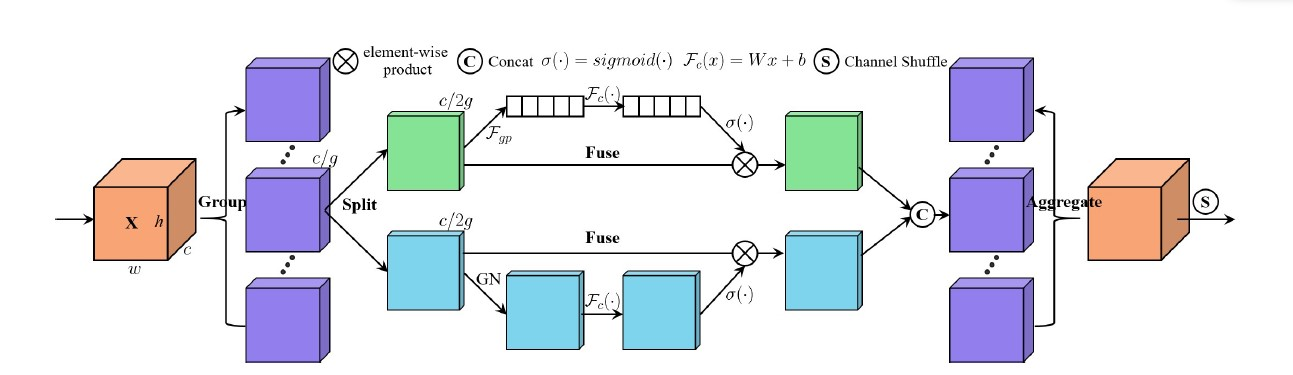• 代码实现
```import torch
from light_cnns import mbv2_sa
model = mbv2_sa()
model.eval()
print(model)
input = torch.randn(1, 3, 224, 224)
y = model(input)
print(y.size())```

#### Triplet attention

• Rotate to Attend: Convolutional Triplet Attention Module

• 网络结构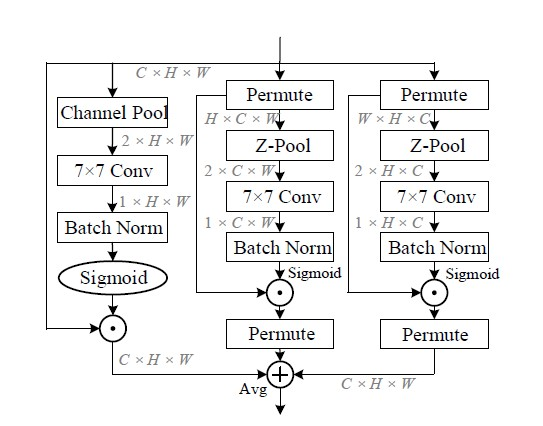• 代码结构
```import torch
from light_cnns import mbv2_triplet
model = mbv2_triplet()
model.eval()
print(model)
input = torch.randn(1, 3, 224, 224)
y = model(input)
print(y.size())```

### 移动端部署CPU网络架构

##### 移动端的最强轻量级网络架构来啦！ LCNet吊打现有主流轻量型网络（附代码实现）

PP-LCNet: A Lightweight CPU Convolutional Neural Network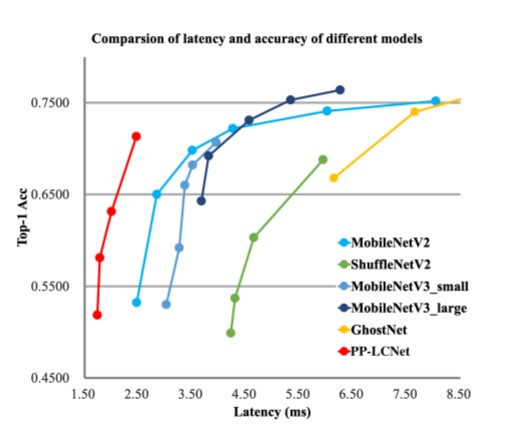• 如何在不增加延迟的情况下促进网络学习到更强的表征能力？
• 目前有哪些要素可以在CPU上提高轻量级模型精度?
• 如何有效结合不同的策略（激活函数、注意力模块、各模块间的位置顺序等等）在CPU上设计出轻量级模型？

#### 代码实现如下：

```import torch
from light_cnns import lcnet_baseline
model = lcnet_baseline()
model.eval()
print(model)
input = torch.randn(1, 3, 224, 224)
y = model(input)
print(y.size())```

### Transformer轻量级网络结构

MobileViT: 一种更小，更快,高精度的轻量级Transformer端侧网络架构

```import torch
from light_cnns import mobilevit_s
model = mobilevit_s()
model.eval()
print(model)
input = torch.randn(1, 3, 224, 224)
y = model(input)
print(y.size())```

### Inception系列

#### Going Deeper with Convolutions (GoogleNet)

1. 首先使用1x1的卷积来进行升降维，这样就巧妙地解决了针对采用较大的卷积核计算复杂度较大这一问题；
2. 然后再在多个不同尺度上运用不同大小的卷积核同时进行卷积，最后将特征进行聚合。```import torch
from light_cnns import googlenet
model.eval()
print(model)
input = torch.randn(1, 3, 224, 224)
y = model(input)
print(y.size())```

#### Rethinking the Inception Architecture for Computer Vision

Inceptionv2针对InceptionV1改进的点主要有：

1. 引入了BN层来对中间特征进行归一化。使用BN层之后，可以加快收敛速度，防止模型出现过拟合.
2. 使用因子分解的方法，主要包括：将 5x5 的卷积分解为两个 3x3 的卷积运算以提升计算速度；将 nxn 的卷积核尺寸分解为 1xn 和 nx1 两个卷积.
3. 扩展模型的宽度，来有效地解决表征性瓶颈问题。

```import torch
from light_cnns import inception_v2
model = inception_v2()
model.eval()
print(model)
input = torch.randn(1, 3, 224, 224)
y = model(input)
print(y.size())```

Inception Net v3 整合了前面 Inception v2 的特点，除此之外，还包括以下5点改进：

1. 不再直接使用max pooling层进行下采样，作者设计了另外一种方案，即两个并行的分支，一个是pooling层，另外一个卷积层，最后将两者结果concat在一起。这样在使用较小的计算量情形下还可以避免瓶颈层，ShuffleNet中也采用了这种策略。

2. 使用RMSProp 优化器；

3. Factorized 7x7 卷积；

4. 辅助分类器使用了 BatchNorm；

5. 使用了label smoothing;

```import torch
from light_cnns import inception_v3
model = inception_v3()
model.eval()
print(model)
input = torch.randn(1, 3, 224, 224)
y = model(input)
print(y.size())```

#### Inception-v4, Inception-ResNet and the Impact of Residual Connections on Learning

1. Inception v4 引入了一个新的stem模块，该模块放在Inception块之间执行。
2. 基于新的stem和Inception 模块，Inception v4重新提出了三种新的Inception模块分别称为 A、B 和 C
3. 引入了专用的「缩减块」（reduction block），它被用于改变网格的宽度和高度。
```import torch
from light_cnns import inception_v4
model = inception_v4()
model.eval()
print(model)
input = torch.randn(1, 3, 224, 224)
y = model(input)
print(y.size())```

#### Xception: Deep Learning with Depthwise Separable Convolutions

1. 将Inception模块简化，仅保留包含3x3的卷积的分支：
2. 将所有1x1的卷积进行拼接.
3. 进一步增加3x3的卷积的分支的数量，使它与1x1的卷积的输出通道数相等：
4. 此时每个3x3的卷积即作用于仅包含一个通道的特征图上，作者称之为“极致的Inception（Extream Inception）”模块，这就是Xception的基本模块。事实上，调节每个3x3的卷积作用的特征图的通道数， 即调节3x3的卷积的分支的数量与1x1的卷积的输出通道数的比例，可以实现一系列处于传统Inception模块和“极致的Inception”模块之间的状态。

```import torch
from light_cnns import xception
model = xception()
model.eval()
print(model)
input = torch.randn(1, 3, 224, 224)
y = model(input)
print(y.size())```

#### Inception Convolution with Efficient Dilation Search（CVPR2021 oral）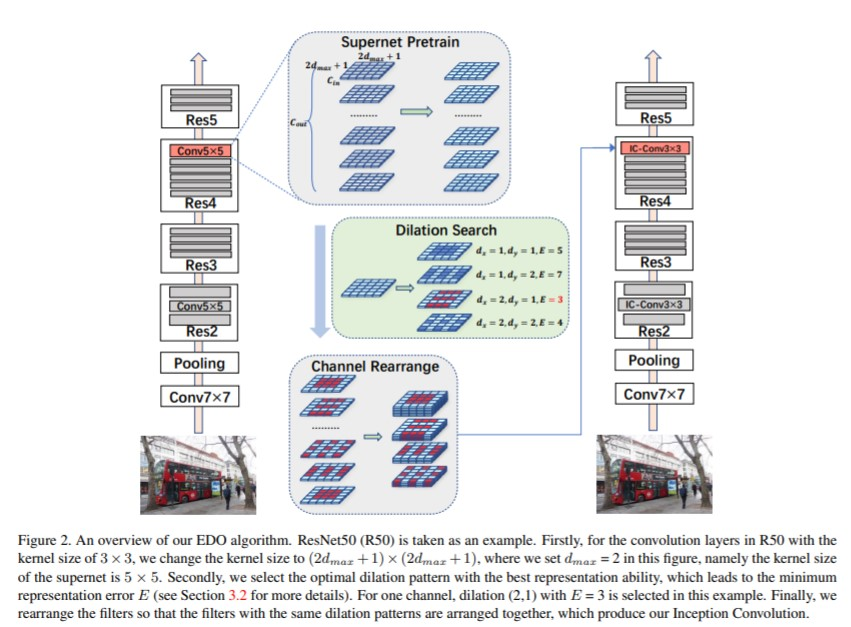```import torch
from light_cnns import ic_resnet50
patter = './pattern_zoo/detection/ic_resnet50_k9.json'
model = ic_resnet50(pattern_path=patter)
model.eval()
print(model)
input = torch.randn(1, 3, 224, 224)
y = model(input)
print(y.size())```

### 轻量级图像分割网络架构

#### ESPNet系列：自动驾驶领域轻量级分割模型

ESPNet: Efficient Spatial Pyramid of Dilated Convolutions for Semantic Segmentation

ESPNet是用于语义分割的轻量级网络，主要提出一种高效空间金字塔卷积模块（ESP Module），该模块包含point-wise卷积和空洞卷积金字塔,有助于减小模型运算量和内存、功率消耗，以提高在终端设备上的适用性。综合比较，ESPNet能在GPU/笔记本/终端设备上达到112FPS/21FPS/9FPS。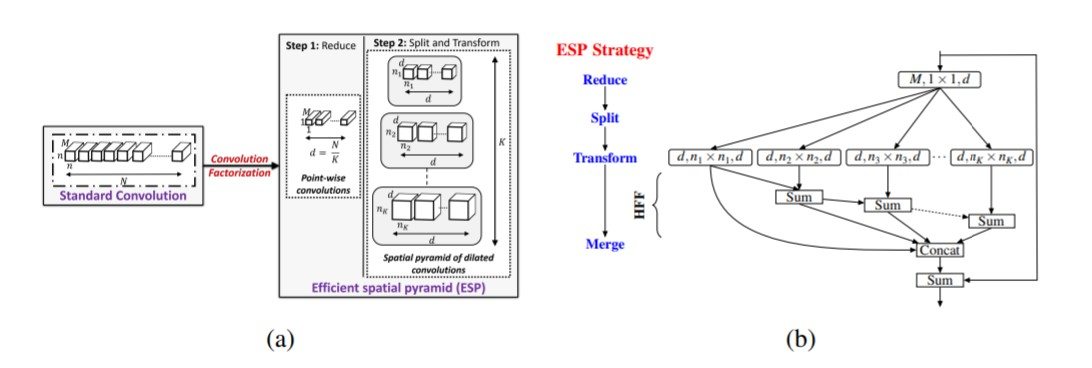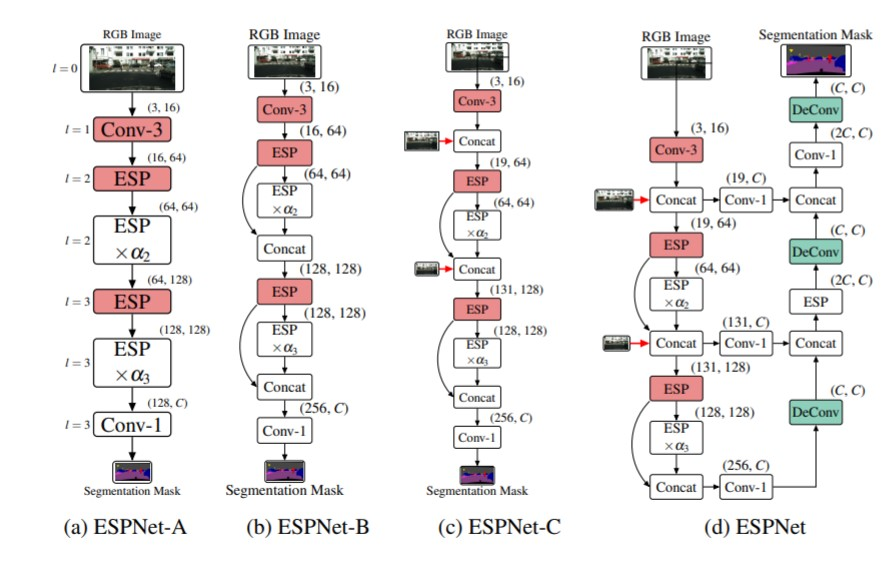```import torch
from light_cnns import espnetv1
model = espnetv1()
model.eval()
print(model)
input = torch.randn(1, 3, 224, 224)
y = model(input)
print(y.size())```

#### ESPNetv2: A Light-weight, Power Efficient, and General Purpose Convolutional Neural Network

ESPNetv2主要基于ESPNetv1进行了模型轻量化处理，主要包括：

1. 基于深度可分离空洞卷积以及分组point-wise卷积改进ESP模块，提出了EESP(Extremely Efficient Spatial Pyramid)模块。相对于ESPNet拥有更好的精度以及更少的参数。

2. 设计了cyclic learning rate scheduler，比一般的固定学习率的scheduler要好。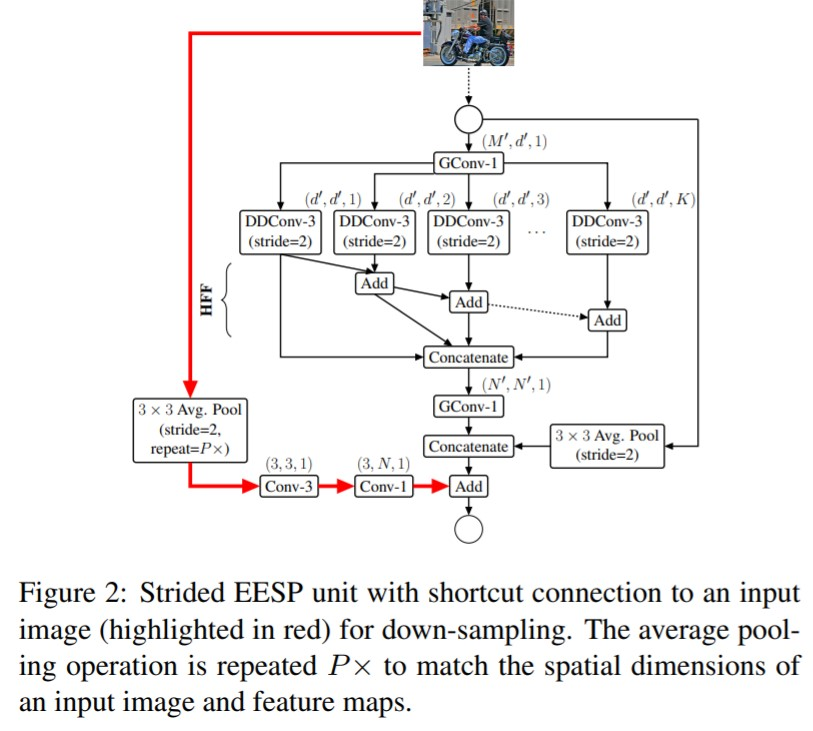```import torch
from light_cnns import espnetv2
model = espnetv2()
model.eval()
print(model)
input = torch.randn(1, 3, 224, 224)
y = model(input)
print(y.size())```